• 摘要：目的：三维分割是医学图像分析和可视化中的重要组成部分，也是医学图像分割中的一个难点。水平集方法在三 ...实现了快速的三维医学图像分割。 关键词：水平集；医学图像分割；CUDA；并行图像处理
• 提出一种交互式的三维医学图像分割算法。结合 Otsu单阈值图像分割算法,提出了一种基于类别方差的双阈值分割算法。利用该算法对三维医学图像体数据的直方图进行了分析,最终得到的上下阈值使得分割结果具有最大类间...
• 借鉴模拟退火思想对三维医学图像的最佳熵函数进行拉伸，构造出改进的遗传算法适应度函数，同时采用精英选择策略保留各代最优个体以加快算法收敛速度，从而提出了一种新的基于多阈值最佳熵的三维医学图像分割遗传搜索...
• 医学图像分割后，如何消除噪声，我采用了去除孤立点的方法。先找到分割结果中的所有连通域，保留最大连通域或者较大的几个连通域，把其余小连通域都去掉。图像是三维的，还蛮有效果的。 import nibabel as nib ...
医学图像分割后，如何消除噪声，我采用了去除孤立点的方法。先找到分割结果中的所有连通域，保留最大连通域或者较大的几个连通域，把其余小连通域都去掉。图像是三维的，还蛮有效果的。
import nibabel as nib
import numpy as np
import os
from skimage import measure

def file_name(file_dir):
L=[]
path_list = os.listdir(file_dir)
path_list.sort() #对读取的路径进行排序
for filename in path_list:
if 'nii' in filename:
L.append(os.path.join(filename))
return L

def Dice(y_true, y_pred):
y_true = y_true
y_pred = y_pred
y_true_f = y_true.flatten()
y_pred_f = y_pred.flatten()
intersection = np.sum(y_true_f * y_pred_f)
return (2. * intersection + 1) / (np.sum(y_true_f) + np.sum(y_pred_f) + 1)

gtnames = ''
prednames = ''

labels_num = np.zeros(len(prednames))
NUM=[]
P=[]

dice_y = np.zeros(len(prednames))
dice_p = np.zeros(len(prednames))
for i in range(len(prednames)):

labels = pred.get_data()
labelsy = np.copy(labels)

labels = measure.label(labels, connectivity=2)

max_num = 0
for j in range(1, np.max(labels)+1):
if np.sum(labels==j) > max_num:
max_num = np.sum(labels==j)
max_pixel = j
print(np.sum(labels==j), np.sum(labels!=0))
if np.sum(labels==j)>0.1*np.sum(labels!=0):
labels[labels==j] = max_pixel

labels[labels != max_pixel]=0
labels[labels == max_pixel]=1
#    labels[labels>1]=0

print(dice_y[i], dice_p[i])
nib.save(nib.Nifti1Image( labels.astype('uint8'), affine=gt.affine), gtnames[i])
代码是针对我做的具体图像写的，可能有些冗余的地方。关键在于labels = measure.label(labels, connectivity=2) 这行代码，需要深入了解的小伙伴可以看下measure函数的用法。
展开全文最大连通域
• 1 基本思想： 令（n+1)函数 t = phi(x(t)) 等于0，即水平集。 2 基本问题： 2.1 初始化 定义 level set 函数 phi(x) 为符号距离函数，界面线上距离为0。一般为隐函数。 2.1.1 符号判定， 界面闭合线内/外 2.1.1.1 ...
一、简介
1 基本思想： 令（n+1)维函数 t = phi(x(t)) 等于0，即水平集。
2 基本问题： 2.1 初始化 定义 level set 函数 phi(x) 为符号距离函数，界面线上距离为0。一般为隐函数。 2.1.1 符号判定， 界面闭合线内/外 2.1.1.1 2D留数算法： 将边界C离散成n段，c_1, c_2, … c_n 为结点， oc_i 表示某点o 指向c_i的向量 sum ( atan(( oc_i x oc_(i+1)) / (oc_i . oc_(i+1))), i = 1: n) if sum == 0 —> 点o 在边界C外 if sum == 2 * pi —> 点o在边界C内 2.1.1.2 交点判定算法: 给定已知符号的点P0, 待定符号的点Px 与 P0 之间连线穿过边界C， 如果穿过次数是偶数表示Px与P0符号相同； 如果是奇数，则符号相反。 实现： 将边界C离散成n段，初始定义所有网格点（2D面）符号为正（C外），当离散的边界线段，与某条垂向网格线相交，则将该网格线上，在边界线段上侧的点全部变负号。 2.1.2 距离计算 2.1.2.1 边界C可由参数方程表示，先搜索距离边界线最近的网格点，计算距离； 2.1.2.2 边界C分段连续，直接计算各个端点到网格点的距离 2.2 演化方程 2.2.1 边界线随时间的变化，采用PDE描述 phi(x(t), t) = 0 d phi(x)/ dt + grad_phi (a. n + b.t) = 0 其中n是法向单位向量，t是切向单位向量。a是法向速度，b是切向速度。n影响边界线拓扑。 <- - n = grad_ phi / norm( grad_phi) –> d phi(x)/ dt = a norm(grad_phi) = 0 2. 2.2 演化方程的数值解 演化方程具有 Hamilton-Jacobi形式， d phi /dt + H(grad_phi) = 0 。 这类方程不存在经典解，只有弱解。解C0连续但不保证C1连续，即使初值C1连续。守恒型双曲PDE也有类似的解性质。比如超声速压缩流动，给定连续的初状态值，仍然可能演化出不连续的间断激波。所以演化方程的高阶精度数值解可借鉴守恒型双曲方程的ENO， WENO等解方案。LSM演化方程实际上是就是一个包含源项的守恒型双曲方程。 常用一阶精度的演化方程常采用迎风格式，Godunov格式， Lax_Friedrich格式等。 2.2.2.1 2D Godunov格式 norm(grad_phi) ~= ( max(-D_{+x}，D_{-x}, 0) ^2 + max(-D_{+y}, D_{-y}, 0)^2 ) ^.5; D_{+x} forward差分, D_{-x} backward差分。同理 D_{+y}, D_{-y}。 物理意义上要求保证信息必须从已知传向未知，所以对于每个当前要求的phi， 必须选择传播正方向上的差分格式。 一个简单的判断：当地(local) phi_x (i,j) 大于还是小于0. 如果 大于0，表示phi(i,j)沿x轴正方向传播，显然先要经过phi(i-1, j)，故应该选用 D_{-x}差分。而且此时还满足 abs( D_{-x}) > abs(D_{+x})， 所以才有统一的上式样子。同理，如果local phi_x(i,j) 小于0， 则表示 phi(i,j )沿x轴负方向传播，即先经过 phi(i+1, j)， 故采用 D_{+x}。同理y方向。 MATALB CODE here 2. 2.2.2 ENO格式 参见 力学所李新亮老师CFD讲义， 激波平滑。
2.2 法向速度延扩 从边界线到全水平集面，任意垂直边界线的直线上速度恒定 --》 所以水平集面上任意一点的速度，可由两条垂直边界线的直线l1, l2唯一确定
2.3 边界设定 布置ghost point， 速度边界Ok, 位移边界可能出问题 – > 重初始化(重新选择一个level set funtion) 假定周期边界 --》 保证单元边界符号连续
2.3 窄带level set method 只跟新距离边界线一定范围的网格点，远场点保持不变。节省计算/存储。
二、源代码
unction [phi L_z] = ls_sparse(phi, L_z, h, iter)
% Whitaker "A Level-Set Approach to 3D Reconstruction..." IJCV 1998
CFL = 0.15;
% shift and peek operations
peekD = @(M,rr,cc) M(safe_sub2ind(size(phi), rr-1, cc));
peekU = @(M,rr,cc) M(safe_sub2ind(size(phi), rr+1, cc));
peekR = @(M,rr,cc) M(safe_sub2ind(size(phi), rr,   cc-1));
peekL = @(M,rr,cc) M(safe_sub2ind(size(phi), rr,   cc+1));
shiftD = @(M) M([1 1:end-1],:);
shiftL = @(M) M(:,[2:end end]);
shiftR = @(M) M(:,[1 1:end-1]);
shiftU = @(M) M([2:end end],:);

%- gather layers
Nd = shiftD(phi); Nu = shiftU(phi); Nr = shiftR(phi); Nl = shiftL(phi);
L_i = find(phi < -.5 & (Nu >= -.5 | Nd >= -.5 | Nl >= -.5 | Nr >= -.5));
L_o = find(phi >  .5 & (Nu <=  .5 | Nd <=  .5 | Nl <=  .5 | Nr <=  .5));
%- layer reverse lookup
L_rev = 2*ones(size(phi), 'int8'); % default: outside
L_rev(phi < 0) = -2; % inside
L_rev(L_i) = -1;
L_rev(L_o) =  1;
L_rev(L_z) =  0;

for i = 1:iter
% record sign
L_z_ = L_z;
L_z_phi_ = phi(L_z);

%- 1. update value of zero layer (with uncontrollable force)
F = h.init_iteration(phi, L_z);
phi(L_z) = phi(L_z) - CFL*F/max(abs(F)); % control that force

%- 3. update status of zero layer
S_i = L_z(phi(L_z) < -.5     );
S_o = L_z(      .5 < phi(L_z));
L_z(phi(L_z) < -.5 | .5 < phi(L_z)) = []; % drop

%- 2. update value of nonzero layers
[rr cc] = ind2sub(size(phi), L_i);
N = [peekR(phi,rr,cc) peekL(phi,rr,cc) peekU(phi,rr,cc) peekD(phi,rr,cc)]';
phi(L_i) = max(N) - 1;
[rr cc] = ind2sub(size(phi), L_o);
N = [peekR(phi,rr,cc) peekL(phi,rr,cc) peekU(phi,rr,cc) peekD(phi,rr,cc)]';
phi(L_o) = min(N) + 1;

%- 4. update status of nonzero layers
S_zi = L_i(     -.5 <= phi(L_i));
S_zo = L_o(phi(L_o) <= .5      );
L_rev(L_i(phi(L_i) < -1.5)) = -2;
L_rev(L_o(phi(L_o) >  1.5)) =  2;
L_i(phi(L_i) < -1.5 | -.5 <= phi(L_i)) = [];
L_o(phi(L_o) <=  .5 | 1.5 <  phi(L_o)) = [];

%- 5. update reverse lookup
L_rev(S_i) = -1;
L_rev(S_o) =  1;
L_rev([S_zi; S_zo]) = 0;

Nl = shiftL(L_rev); Nr = shiftR(L_rev);
Nu = shiftU(L_rev); Nd = shiftD(L_rev);
is_near = Nl==0 | Nr==0 | Nu==0 | Nd==0;
S_oo = find(L_rev ==  2 & is_near);
S_ii = find(L_rev == -2 & is_near);
L_o = [L_o; S_o; S_oo];
L_i = [L_i; S_i; S_ii];
L_z = [L_z; S_zi; S_zo];
L_rev(S_ii) = -1;
L_rev(S_oo) =  1;

%- 8. update values of new neighbors
[rr cc] = ind2sub(size(phi), S_ii);
N = [peekR(phi,rr,cc) peekL(phi,rr,cc) peekU(phi,rr,cc) peekD(phi,rr,cc)]';
phi(S_ii) = max(N) - 1;
[rr cc] = ind2sub(size(phi), S_oo);
N = [peekR(phi,rr,cc) peekL(phi,rr,cc) peekU(phi,rr,cc) peekD(phi,rr,cc)]';
phi(S_oo) = min(N) + 1;

%- 9. update bookkeeping
L_z_phi = phi(L_z_);
idx_out = L_z_(L_z_phi_ >= 0  & L_z_phi < 0);
idx_in  = L_z_(L_z_phi_ < 0 & L_z_phi >= 0);
h.move_in(idx_in);
h.move_out(idx_out);
end
end
function [phi L_z] = ls_sparse3(phi, L_z, h, iter,img)
% Whitaker "A Level-Set Approach to 3D Reconstruction..." IJCV 1998
CFL = .4;
% shift and peek operations
peekD = @(M,rr,cc,dd) M(safe_sub2ind(size(phi), rr-1, cc,   dd));
peekU = @(M,rr,cc,dd) M(safe_sub2ind(size(phi), rr+1, cc,   dd));
peekR = @(M,rr,cc,dd) M(safe_sub2ind(size(phi), rr,   cc-1, dd));
peekL = @(M,rr,cc,dd) M(safe_sub2ind(size(phi), rr,   cc+1, dd));
peekF = @(M,rr,cc,dd) M(safe_sub2ind(size(phi), rr,   cc,   dd+1));
peekB = @(M,rr,cc,dd) M(safe_sub2ind(size(phi), rr,   cc,   dd-1));
shiftD = @(M) M([1 1:end-1],:,:);
shiftL = @(M) M(:,[2:end end],:);
shiftR = @(M) M(:,[1 1:end-1],:);
shiftU = @(M) M([2:end end],:,:);
shiftF = @(M) M(:,:,[2:end end]);
shiftB = @(M) M(:,:,[1 1:end-1]);

%- gather layers
Nd = shiftD(phi); Nu = shiftU(phi); Nr = shiftR(phi); Nl = shiftL(phi); Nf = shiftF(phi); Nb = shiftB(phi);
L_i = find(phi < -.5 & (Nu >= -.5 | Nd >= -.5 | Nl >= -.5 | Nr >= -.5 | Nf >= -.5 | Nb >= -.5));
L_o = find(phi >  .5 & (Nu <=  .5 | Nd <=  .5 | Nl <=  .5 | Nr <=  .5 | Nf <=  .5 | Nb <=  .5));
%- layer reverse lookup
L_rev = 2*ones(size(phi), 'int8'); % default: outside
L_rev(phi < 0) = -2; % inside
L_rev(L_i) = -1;
L_rev(L_o) =  1;
L_rev(L_z) =  0;

for i = 1:iter
% record sign
L_z_ = L_z;
L_z_phi_ = phi(L_z);

%- 1. update value of zero layer (with uncontrollable force)
F = h.init_iteration(phi, L_z,img);%speed1%speed2
%     F = h.init_iteration(phi, L_z);%mean throshhold..
phi(L_z) = phi(L_z) - CFL*F/max(abs(F)); % control that force

%- 3. update status of zero layer
S_i = L_z(phi(L_z) < -.5     );
S_o = L_z(      .5 < phi(L_z));
L_z(phi(L_z) < -.5 | .5 < phi(L_z)) = []; % drop

%- 2. update value of nonzero layers
[rr cc dd] = ind2sub(size(phi), L_i);
N = [peekR(phi,rr,cc,dd) peekL(phi,rr,cc,dd) ...
peekU(phi,rr,cc,dd) peekD(phi,rr,cc,dd) ...
peekF(phi,rr,cc,dd) peekB(phi,rr,cc,dd)];
phi(L_i) = max(N,[],2) - 1;
[rr cc dd] = ind2sub(size(phi), L_o);
N = [peekR(phi,rr,cc,dd) peekL(phi,rr,cc,dd) ...
peekU(phi,rr,cc,dd) peekD(phi,rr,cc,dd) ...
peekF(phi,rr,cc,dd) peekB(phi,rr,cc,dd)];
phi(L_o) = min(N,[],2) + 1;

%- 4. update status of nonzero layers
S_zi = L_i(     -.5 <= phi(L_i));
S_zo = L_o(phi(L_o) <= .5      );
L_rev(L_i(phi(L_i) < -1.5)) = -2;
L_rev(L_o(phi(L_o) >  1.5)) =  2;
L_i(phi(L_i) < -1.5 | -.5 <= phi(L_i)) = [];
L_o(phi(L_o) <=  .5 | 1.5 <  phi(L_o)) = [];

%- 5. update reverse lookup
L_rev(S_i) = -1;
L_rev(S_o) =  1;
L_rev([S_zi; S_zo]) = 0;

Nl = shiftL(L_rev) == 0; Nr = shiftR(L_rev) == 0; Nu = shiftU(L_rev) == 0;
Nd = shiftD(L_rev) == 0; Nf = shiftF(L_rev) == 0; Nb = shiftB(L_rev) == 0;
is_near = Nl | Nr | Nu | Nd | Nf | Nb;
S_oo = find(L_rev ==  2 & is_near);
S_ii = find(L_rev == -2 & is_near);
L_o = [L_o; S_o; S_oo];
L_i = [L_i; S_i; S_ii];
L_z = [L_z; S_zi; S_zo];
L_rev(S_ii) = -1;
L_rev(S_oo) =  1;

%- 8. update values of new neighbors
[rr cc dd] = ind2sub(size(phi), S_ii);
N = [peekR(phi,rr,cc,dd) peekL(phi,rr,cc,dd) ...
peekU(phi,rr,cc,dd) peekD(phi,rr,cc,dd) ...
peekF(phi,rr,cc,dd) peekB(phi,rr,cc,dd)];
phi(S_ii) = max(N,[],2) - 1;
[rr cc dd] = ind2sub(size(phi), S_oo);
N = [peekR(phi,rr,cc,dd) peekL(phi,rr,cc,dd) ...
peekU(phi,rr,cc,dd) peekD(phi,rr,cc,dd) ...
peekF(phi,rr,cc,dd) peekB(phi,rr,cc,dd)];
phi(S_oo) = min(N,[],2) + 1;

%- 9. update bookkeeping
L_z_phi = phi(L_z_);
idx_out = L_z_(L_z_phi_ > 0  & L_z_phi <= 0);
idx_in  = L_z_(L_z_phi_ <= 0 & L_z_phi > 0);
h.move_in(idx_in);
h.move_out(idx_out);
end
end

三、运行结果四、备注
版本：2014a
展开全文• 1 基本思想： 令（n+1)函数 t = phi(x(t)) 等于0，即水平集。 2 基本问题： 2.1 初始化 定义 level set 函数 phi(x) 为符号距离函数，界面线上距离为0。一般为隐函数。 2.1.1 符号判定， 界面闭合线内/外 2.1.1.1 ...
一、简介
1 基本思想： 令（n+1)维函数 t = phi(x(t)) 等于0，即水平集。
2 基本问题： 2.1 初始化 定义 level set 函数 phi(x) 为符号距离函数，界面线上距离为0。一般为隐函数。 2.1.1 符号判定， 界面闭合线内/外 2.1.1.1 2D留数算法： 将边界C离散成n段，c_1, c_2, … c_n 为结点， oc_i 表示某点o 指向c_i的向量 sum ( atan(( oc_i x oc_(i+1)) / (oc_i . oc_(i+1))), i = 1: n) if sum == 0 —> 点o 在边界C外 if sum == 2 * pi —> 点o在边界C内 2.1.1.2 交点判定算法: 给定已知符号的点P0, 待定符号的点Px 与 P0 之间连线穿过边界C， 如果穿过次数是偶数表示Px与P0符号相同； 如果是奇数，则符号相反。 实现： 将边界C离散成n段，初始定义所有网格点（2D面）符号为正（C外），当离散的边界线段，与某条垂向网格线相交，则将该网格线上，在边界线段上侧的点全部变负号。 2.1.2 距离计算 2.1.2.1 边界C可由参数方程表示，先搜索距离边界线最近的网格点，计算距离； 2.1.2.2 边界C分段连续，直接计算各个端点到网格点的距离 2.2 演化方程 2.2.1 边界线随时间的变化，采用PDE描述 phi(x(t), t) = 0 d phi
展开全文• 基于MARL (IteR-MRL)迭代优化交互式三维医学图像分割算法综述在每个细化步骤中，将包含图像的状态、先前的分割概率和提示图输入到actor网络中，然后actor网络根据其输出动作生成当前分割概率。接下来，用户根据错误...


论文笔记：Iteratively-Refined Interactive 3D Medical Image Segmentation with Multi-Agent Reinforcement Learning
Abstract1. Introduction2. Related work3. Methodology3.1 Overview3.2. Multi-agent RL framework for interactive im-StateActionReward

3.3. Network and training
4. Experiments4.1. Datasets4.2. SettingsEvaluation metrics.User simulationImplementation details

4.3. Results
5. Conclusion

论文来自：2020年CVPR
作者：Xuan Liao , Wenhao Li , Qisen Xu , Xiangfeng Wang ,Bo Jin , Xiaoyun Zhang , Yanfeng Wang , and Ya Zhang
单位：上海交通大学，华东师范大学
Abstract
现有的三维图像自动分割方法往往不能满足临床应用的需要。许多研究提出了一种通过迭代地结合用户提示来提高图像分割性能的交互式策略。然而，连续交互的动态过程在很大程度上被忽略了。本文提出将迭代交互式图像分割的动态过程建模为马尔可夫决策过程(MDP)，并用强化学习(RL)方法求解。由于勘探空间大，单智能体RL难以进行体素预测。为了将探索空间缩小到可处理的大小，我们将每个体素视为具有共享体素级别行为策略的代理，这样它就可以通过多代理强化学习来解决。这种多主体模型的另一个优点是捕获体素之间的依赖性，用于分割任务。同时，为了丰富先前分割的信息，我们在MDP的状态空间中保留了预测的不确定性，推导了一个调整动作空间，使得分割更精确、更精细。此外，为了提高探索效率，我们设计了相对的基于交叉熵收益的奖励机制，在约束方向上更新策略。对各种医学数据集的实验结果表明，我们的方法显著优于现有的最先进的方法，具有交互更少和收敛更快的优势。
1. Introduction
随着卷积神经网络（CNNs）的发展，自动分割医学图像取得了巨大的进步。但是当前自动方法的准确性和鲁棒性都有待提高。 自动获取粗分割思想：将在初始输入中包含粗分割的方法称为更新方法。目前更新方法存在以下两种主要问题：
通常忽略连续交互的动态过程。虽然分割可以细化，但是该模型总是单独处理每个细化分割的步骤，而不包含先前的信息。另一个问题是预测不确定性的损失，因为是使用二进制分割结果而不是每个体素分割概率作为模型输入。将密集分割概率舍入到二值分割预测可能会造成量化误差和精度损失。
为了解决以上两个问题。 本文提出了一种交互式医学图像分割的更新方法：基于多智能体强化学习的迭代精细交互式三维医学图像分割（IteR-MRL）。将交互式图像分割的动态过程以MDP的形式表述出来。同时，设计了一个基于相对交叉熵增益的奖励机制，以促使智能体更有效地进行探索，而不是考虑当前预测结果与实际情况之间的差异。为了将探索空间缩小到可控制的大小，并明确地建模体素间的依赖关系，我们引入了多智能体的强化学习(MARL)方法
主要贡献：
我们将交互式图像分割任务定义为MDP，并提出了一种基于MARL的基于体素的交互式分割框架，用于三维医学图像，使用户交互得到更有效的利用。提出利用分割概率保留预测不确定性，丰富先前分割的信息，使调整更精确、更精细。量的实验表明，在迭代序列中，考虑两步之间的相对增益，在只有少量交互和快速收敛的情况下，分割效果显著提高。
2. Related work
交互式的图像分割方法广泛引用于自然图像和医学图像中。 以下为现有的一些方法：
Graph-based interactive image segmentation（基于图形的交互式图像分割）CNN-based interactive image segmentation（基于CNN网络的交互式图像分割）RL-based interactive image segmentation（基于强化学习的交互式图像分割）
3. Methodology
我们将交互式图像分割定义为MDP，并提出了一种新的基于MARL的交互式医学图像分割方法，以更有效地开发交互信息。
3.1 Overview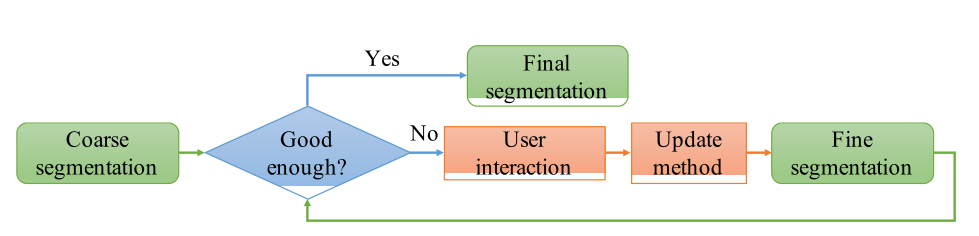图1。迭代细化交互式图像分割方法的流程图。在粗分割条件下，该方法利用用户交互对粗分割进行迭代细化，直到细分割足够好为止。
现有的基于监督学习的算法的主要问题是它们将整个图像细化过程分割成独立的步骤。为了解决这个问题，我们采用RL通过将奖励指定为相对改善来明确捕获成功预测之间的关联。由于体素预测的大状态空间和动作空间以及相互依赖的体素之间的协作的必要性，我们采用MARL的思想:将三维图像中的每个体素视为一个代理。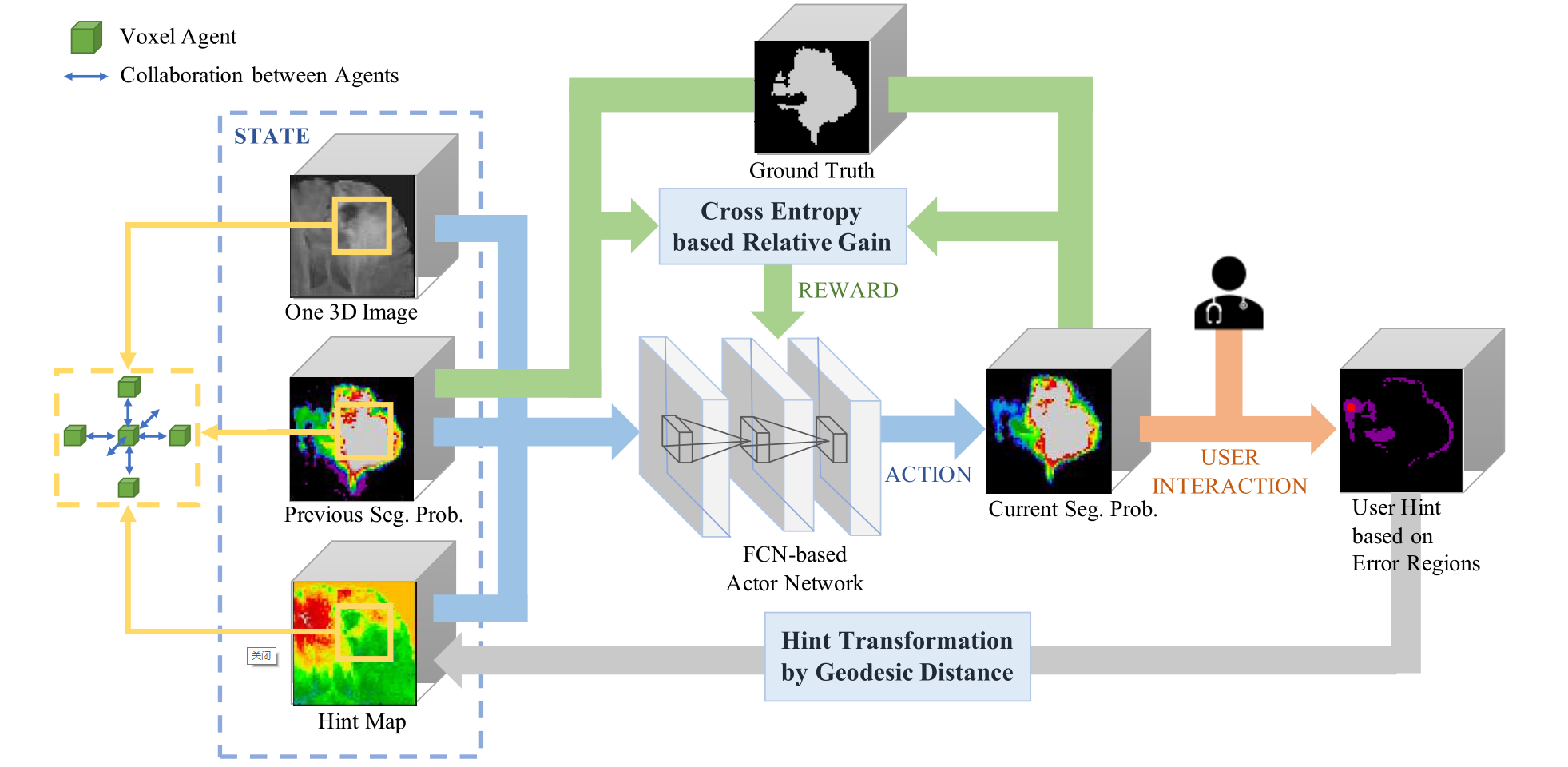图2。基于MARL (IteR-MRL)迭代优化交互式三维医学图像分割算法综述在每个细化步骤中，将包含图像的状态、先前的分割概率和提示图输入到actor网络中，然后actor网络根据其输出动作生成当前分割概率。接下来，用户根据错误区域返回提示点击(红点)，通过提示转换生成新的提示映射。在每一步中，奖励是由之前和当前分割交叉的相对增益决定的。
图片二介绍了本文提出的方法的框架IteR-MRL。中间的actor网络利用原始3D图像、之前的分割概率和交互信息作为状态，对分割概率进行更新生成新的分割概率。动者网络输出调整先前分割概率和产生当前分割概率的智能体参数。之后，对当前分割概率进行两次后续操作。一方面，基于实际情况和逐次分割概率，通过计算当前和之前交叉熵的相对增益，向网络反馈一个奖励信号，用于参数更新。另一方面，它呈现给用户，用户提供反馈，即对预测错误的区域点击物体或背景。图2上的放大显示的红点为用户的点击表示。
3.2. Multi-agent RL framework for interactive im-
age segmentation 本文在此给出了一些MARL设置的描述。设

x

=

(

x

1

,

.

.

.

,

x

N

)

x=(x_1,...,x_N)

是数据集中的任意图像。

x

i

x_i

是x的第i个体素。其中每一个

x

i

x_i

对应的策略都是

π

i

(

a

i

(

t

)

∣

s

i

(

t

)

)

π_i(a_i^{(t)}|s_i^{(t)})

。

s

i

(

t

)

s_i^{(t)}

和

a

i

(

t

)

a_i^{(t)}

表示当前状态，

a

i

(

t

)

∈

A

a_i^{(t)}∈ A

，并且A表示action的集合。

s

i

(

t

)

∈

S

s_i^{(t)}∈ S

，并且S表示state的集合。 整个图像的角度出发，将之前的分割细化为新的分割。通过全局的行动

a

(

t

)

=

(

a

1

(

t

)

,

.

.

.

,

a

N

(

t

)

)

a^{(t)}=(a_1^{(t)},...,a_N^{(t)})

，agent会转换到全局的状态

s

(

t

+

1

)

=

(

s

1

(

t

+

1

)

,

.

.

.

,

s

N

(

t

+

1

)

)

s^{(t+1)}=(s_1^{(t+1)},...,s_N^{(t+1)})

，并且会的到全局的奖励值

r

(

t

)

=

(

r

1

(

t

)

,

.

.

.

,

r

N

(

t

)

)

r^{(t)}=(r_1^{(t)},...,r_N^{(t)})

。
State
对于我们的问题公式，体素代理

x

i

x_i

在步骤 t 处的状态是其体素值

b

i

b_i

的串联，其之前的分割概率

p

i

(

t

)

p_i^{(t)}

为对象标并且它在提示图上的两个值分别为:

h

+

,

i

(

t

)

和

h

−

,

i

(

t

)

h_{+,i}^{(t)} 和h_{-,i}^{(t)}

，所以

s

i

(

t

)

=

[

b

i

,

p

i

(

t

)

,

h

+

,

i

(

t

)

,

h

−

,

i

(

t

)

]

s_i^{(t)}=[b_i,p_i^{(t)},h_{+,i}^{(t)},h_{-,i}^{(t)}]

，其中

p

i

(

t

)

∈

[

0

,

1

]

p_i^{(t)}∈ [0,1]

。在初始状态

s

i

(

0

)

s_i^{(0)}

对应的初始粗糙分割概率定义为

p

i

(

0

)

p_i^{(0)}

。 在讨论整个提示图生成时，在步骤 t 的用户交互过程中，提示的映射是

h

(

t

)

h^{(t)}

是由用户的提示转换而来的，提示又是由用户点击而得到的。提示的数量和位置是根据用户交互规则选择的。 实际上在一张图片中有两个尺寸相同的提示图的通道。对象提示图

h

+

(

t

)

h_+^{(t)}

和背景提示图

h

−

(

t

)

h_-^{(t)}

,它们分别由对象提示集

h

s

+

(

t

)

hs_+^{(t)}

和背景提示集

h

s

−

(

t

)

hs_-^{(t)}

产生。因此，用户提示映射是这两个提示映射的串联:

h

(

t

)

=

[

h

+

(

t

)

,

h

−

(

t

)

]

h^{(t)}=[h_+^{(t)}, h_-^{(t)}]

。对于每一个提示图都有

h

ℓ

t

,

ℓ

∈

{

+

,

−

}

h_ℓ^{t},ℓ ∈ \lbrace+,−\rbrace

，并且定义

h

ℓ

(

t

)

=

(

h

ℓ

,

1

(

t

)

,

.

.

.

,

h

ℓ

,

N

(

t

)

)

h_ℓ^{(t)}=(h_{ℓ,1}^{(t)},...,h_{ℓ,N}^{(t)})

。元素

h

ℓ

,

i

(

t

)

h_{ℓ,i}^{(t)}

在提示图

h

ℓ

(

t

)

h_ℓ^{(t)}

上是由

x

i

x_i

和相应的提示集合

h

s

ℓ

(

t

)

hs_ℓ^{(t)}

之间的最小距离计算得到的：

h

ℓ

,

i

(

t

)

=

m

i

n

∀

x

j

∈

h

s

ℓ

(

t

)

M

(

x

i

,

x

j

)

，

h_{ℓ,i}^{(t)}=min_{ ∀x_j ∈hs_ℓ^{(t)}}M(x_i ,x_j ) ，

其中M是测量两个体素之间距离的函数。两个体素之间的距离是连接这两个体素的所有路径的颜色梯度之和的最小值。（具体看图片2）
Action
为了使调整结果更稳定，不发生突变，我们在此根据之前的概率预估调整量。动作

a

i

(

t

)

∈

A

a_i^{(t)}∈ A

中

x

i

x_i

在第 t 步中通过一个确定的数量

a

i

(

t

)

a_i^{(t)}

来调整之前的分割概率

p

i

(

t

)

p_i^{(t)}

。因此在执行动作

a

i

(

t

)

a_i^{(t)}

后的分割概率为

p

i

(

t

+

1

)

p_i^{(t+1)}

：

p

i

(

t

+

1

)

=

C

0

1

(

p

i

(

t

)

+

a

i

(

t

)

)

，

p_i^{(t+1)}=C_0^1(p_i^{(t)}+a_i^{(t)}) ，

这里

C

a

b

(

x

)

=

m

i

n

(

m

a

x

(

x

,

a

)

,

b

)

C_a^b(x)=min(max(x,a),b)

是将 x 的值从 a 剪切到 b 。其中

p

i

(

t

+

1

)

p_i^{(t+1)}

为一个概率，所以属于 [0,1] 。动作集合

A

=

A

k

(

k

=

1

,

2

,

.

.

.

,

K

)

A={A_k} (k=1,2,...,K)

包含了K个动作。
Reward
为了提高勘探效率，我们设计了一个相对交叉熵收益的奖励机制，在约束方向上更新模型。具体地说，该方法是对先前分割方法的相对改进，在真实情况

y

i

y_i

与分割概率

p

i

p_i

之间的交叉熵

x

i

x_i

的减少:

r

i

(

t

)

=

X

i

(

t

−

1

)

−

X

i

(

t

)

，

（

3

）

r_i^{(t)}=X_i^{(t-1)}-X_i^{(t)} ， （3）

X

i

(

t

)

=

−

y

i

l

o

g

(

p

i

(

t

)

)

−

(

1

−

y

i

)

l

o

g

(

1

−

p

i

(

t

)

)

.

（

4

）

X_i^{(t)}=-y_ilog(p_i^{(t)})-(1-y_i)log(1-p_i^{(t)}). （4）

对于(3)，当agent的概率更接近真正的体素标签时，agent将获得正奖励，反之亦然。相对增益不是一个遥远的目标，而是提供给主体一个比较和超越的基准。 一般来说，一个互动体验的累积回报是：

R

i

=

∑

t

=

1

T

γ

(

t

−

1

)

r

i

(

t

)

，

（

5

）

R_i=\sum_{t=1}^T{γ^{(t-1)}r_i^{(t)}}， （5）

其中T是总步数，折扣因子

γ

∈

(

0

,

1

]

γ∈(0,1]

。
3.3. Network and training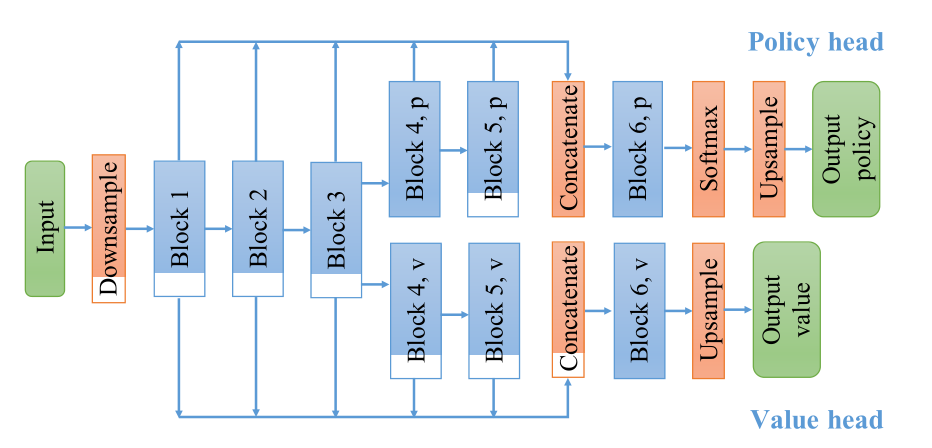图3。IteR-MRL的网络架构。IteR-MRL的网络架构。政策头和价值头共享低级特征，并提取自己的高级特征。 我们分别用

θ

p

θ_p

和

θ

v

θ_v

表示策略的参数和头值。 网络的输入是时间步长 t :

s

(

t

)

s ^{(t)}

处的状态。头值输出当前状态 V (s(t)) 的估计值。θv 的梯度计算公式为:

d

θ

v

=

∇

θ

v

A

(

s

(

t

)

,

a

(

t

)

)

2

,

(

6

)

dθ_v=∇_{θ_v}A(s^{(t)},a^{(t)})^2 , (6)

A

(

s

(

t

)

,

a

(

t

)

)

=

∑

k

=

t

T

γ

k

−

t

r

ˉ

(

k

)

−

V

(

s

(

t

)

)

,

(

7

)

A(s^{(t)},a^{(t)})=\sum_{k=t}^Tγ^{k-t}\bar{r}^{(k)}-V(s^{(t)}) , (7)

其中

r

ˉ

(

k

)

\bar{r}^{(k)}

是时间步长k处所有体素的平均回报。

A

(

s

(

t

)

,

a

(

t

)

)

A(s^{(t)},a^{(t)})

是在第t步的状态

s

(

t

)

s^{(t)}

时采取行动

a

(

t

)

a^{(t)}

时才获得的利益，这表示了不受状态影响的实际累计报酬，降低了梯度方差。策略头输出操作策略：

π

i

(

a

i

(

t

)

∣

s

i

(

t

)

)

π_i(a_i^{(t)}|s_i^{(t)})

，这也就是每次行动

a

(

t

)

a^{(t)}

的概率。

θ

p

θ_p

的梯度由以下公式计算:

d

θ

p

=

−

∇

θ

p

π

(

a

(

t

)

∣

s

(

t

)

)

A

(

a

(

t

)

,

s

(

t

)

)

.

(

8

)

dθ_p=-∇_{θ_p}π(a^{(t)}|s^{(t)})A(a^{(t)},s^{(t)}). (8)

这两个头部以端对端的方式共同进行训练。
4. Experiments
4.1. Datasets
在本文中，我们在三个三维MRI数据集上做实验。每张图像在使用前都是基于其非零坐标进行裁剪的。初始化方法被定义为产生初始化产物的分割方法。如果在更新方法中再使用经过初始方法训练的图像，粗分割概率(更新方法的初始分割可能性)会因为这些图像已经在初始方法中看到了真实情况而过于完美而无法在更新方法中进行细化。因此，我们提出了一种新的数据集分割方法:数据集分为三个部分，两个具有相等输入量的训练集和一个测试集。
具体来说，我们是随机选取的 Ntrain1 个案例作为训练集，形成初始方法的训练集 Dtrain ，然后在剩余的数据集中随机选择另外 Ntrain 个训练集作为更新方法的训练集 Dtrain2。剩下的 Ntest 个测试集作为训练集 Dtest 。 三个数据集如下:
BraTS2015MM-WHSNCI-ISBI2013
4.2. Settings
Evaluation metrics.
通常，医学图像分割是通过骰子分数来评估的:

D

i

c

e

(

S

p

,

S

g

)

=

2

∣

S

p

∩

S

g

∣

∣

S

p

∣

+

∣

S

g

∣

,

(

9

)

Dice(S_p,S_g)= \frac{2|S_p\cap S_g|}{|S_p|+|S_g|},(9)

这里Sp为预测值，Sg为真实值。| · |代表这个区域的体素数量是多少。 在研究交互式图像分割任务时，不仅考虑了骰子分数，而且还考虑了用户的点击次数。我们的目标是用更少的用户点击获得更高的骰子分数。
User simulation
由于需要大量的人力资源来与真实的医生一起进行实验，我们像其他工作一样模拟用户的点击。以往的工作中，训练的点击量多(≈40)，测试的点击量少，而我们的培训和测试的互动策略是一致的。因此，训练设置与测试设置相似，以减少训练与测试之间的偏差。
Implementation details
在预处理过程中，所有图像均按整个数据集的平均值和标准变量进行归一化处理，整个数据集 D = [ Dtrian1, Dtrain2, Dtest ]。
每个图像由边界框裁剪，基于其非零区域，扩展为[0,10]体素，然后将大小调整为55×55×30。数据增强-在三个方向翻转和随机旋转角度范围[-π/8，π/8]在三个方向。
对于不同的数据集，使用Nvidia Titan X GPU的模型训练时间从几小时到两天不等。每个更新步骤的平均推理时间为894ms，其中交互模拟时间为424ms。
4.3. Results
为了进行公平的比较，我们将denseCRF应用到所有与CRF兼容的模上作为最终的精化处理。
Comparisons with state-of-the-art methods 我们用三种最先进的方法来比较IteR-MRL:Min-Cut，DeepIGeoS 和 InterCNN 。 在表1中，更新方法从四种不同的初始分割方法得到粗集:
BG (set all voxel labels to background),V-NetHighRes3DNetDeepIGeoS
实验结果表明，在每种初始方法下，IteR-MRL都比基线获得了更好的结果，说明了该方法的鲁棒性和泛化性更强。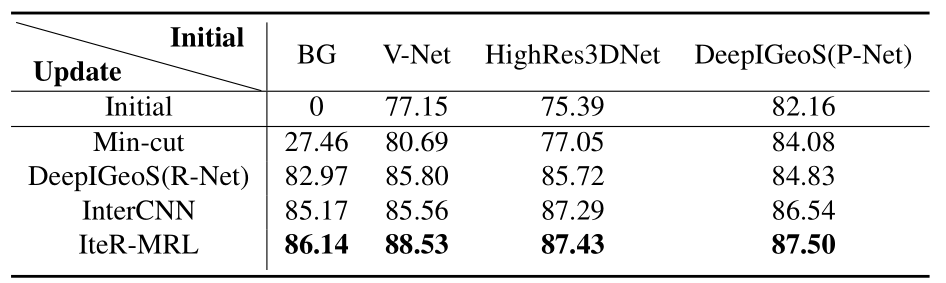表1。结合不同的初始方法
为了验证考虑连续预测间的相对增益是否能导致快速改进，我们还分析了表2中一个改进序列所带来的性能改进。
我们在这里使用V-Net作为初始方法(77.15)。对于第一个优化步骤，所有更新方法的性能都有显著提高(从+2.37提高到+8.47)。从第二步开始，大多数性能都遇到了停滞(非常小的改善)，尽管添加了新的用户提示。DeepIGeoS(R-Net)在第三步甚至有退化(0.01)。其他方法在每一步的细化过程中都有较慢的改进，而IteR-MRL有较高的改进，证明了考虑连续预测之间的关系增益是有效的。每个细化步骤都有很大的改进，最终也获得了良好的结果(88.53)。此外，我们注意到IteR- MRL在第二步的性能已经超过了其他的最终性能，实现了用户点击次数的减少。图4提供了一个相互作用序列中产能改进的全局视图。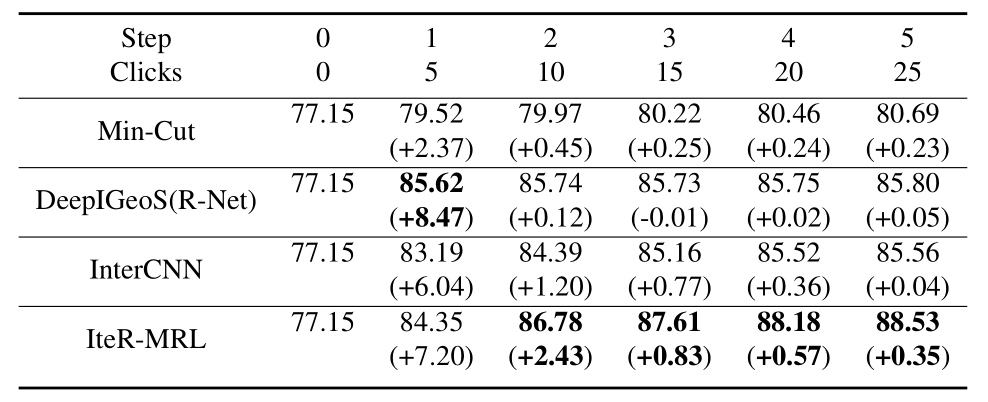表2。在一个交互序列里的性能改善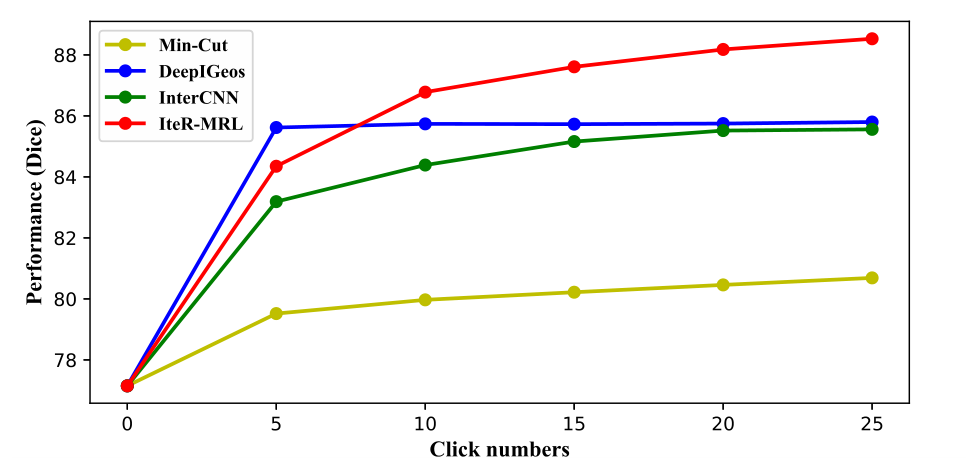图4。性能改进显示曲线
图5给出了以V-Net作为初始方法的不同更新方法的可视化结果。具体地说，我们在五个细化步骤之后可视化地细化细分。可以观察到，其他方法倾向于产生一个相当光滑的边界，而IteR-MRL在捕获边缘细节方面表现得更好。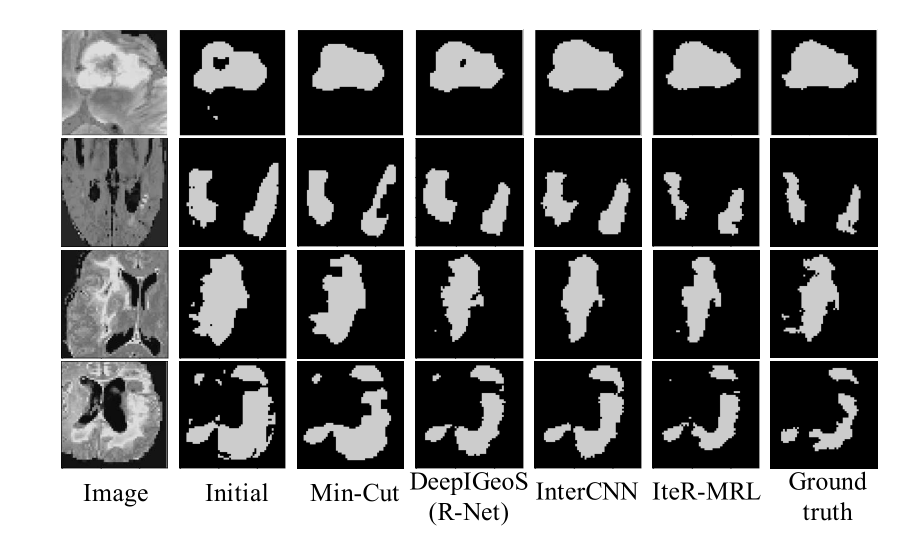图5。不同更新方法的可视化
以上结果是使用BraTS2015数据集进行实验得到的。在MM-WHS和NCI-两个数据集上进行了更多的实验。表3中的ISBI2013对鲁棒性进行了验证，采用初始方法V-Net。结果表明，IteR-MRL在不同类型的数据集上具有稳定的性能。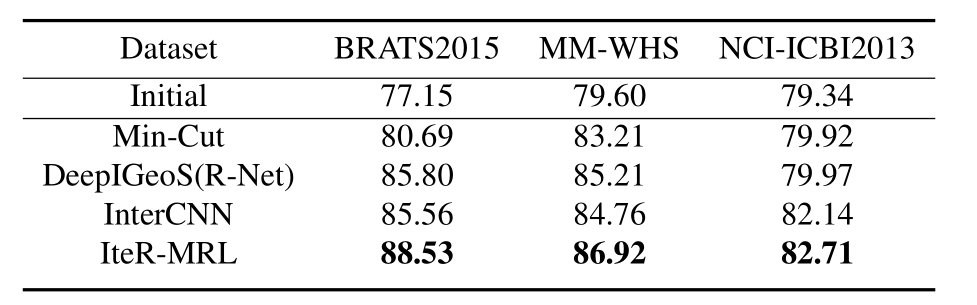表3。在不同数据集上的性能
Ablation study 我们分析了不同动作集对算法性能的影响，见表4。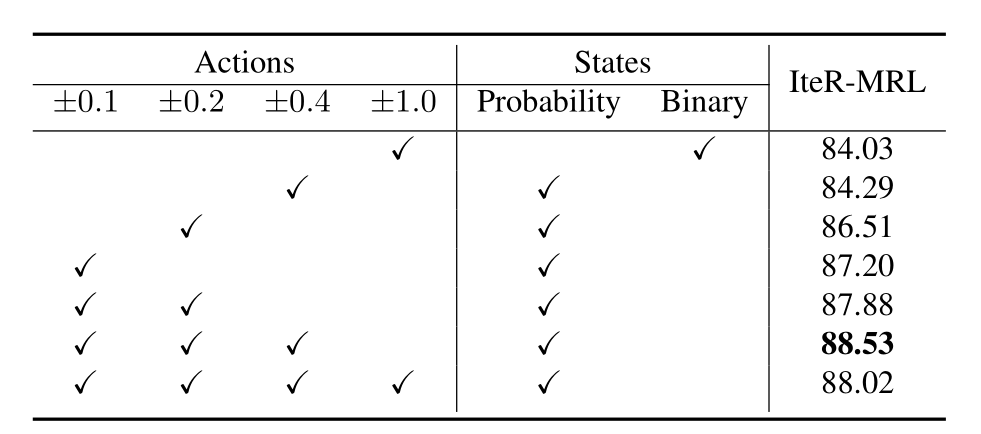表4。不同动作和状态设置的组合
在表5中，我们比较了不同的奖励函数设计。相对奖励的另一种选择是绝对奖励，即当前预测与基础事实之间的差异。结果表明，相对的方法具有更好的性能。一个可能的原因是相对增益能更好地反映agent对分割概率的调整。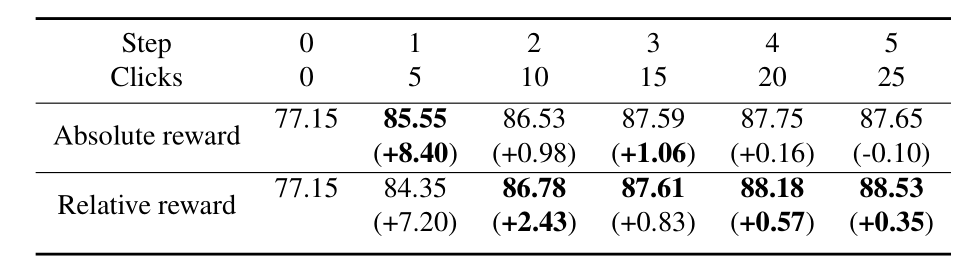表5。奖励函数对性能的影响
交互和模型都能促进性能的提高。现在我们通过改变交互策略来分析交互对性能的贡献有多大。除了前面使用的良好交互外，表6中还做了两个对比实验。没有交互作用的人总是用随机噪声填充提示地图，这样模型就不会接收到任何新的交互信息。另一种交互性较差的方法是在所有体素中随机选择用户点击点。在这种情况下，交互可能向模型传递错误的消息。因此，我们发现有意义的交互确实有助于极大地提高性能。
还可以观察到，具有输出相互作用的模型仍然有一些性能的提高，这可能来自于迭代模型本身。此外，交互不好的交互的退化表明无效的交互会损害性能。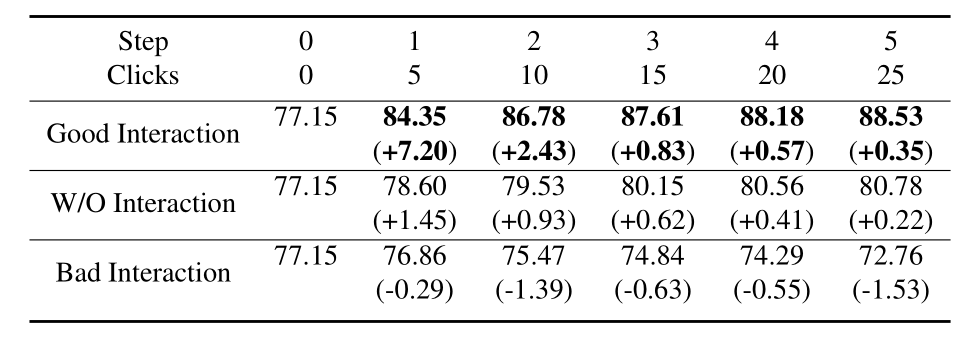表6。交互对性能的贡献
为了验证累积奖励相对于即时奖励的有效性，我们分析了一个训练优化序列的总步数T。表7显示，累积奖励优于即时奖励，即时奖励在多步骤互动中表现较差。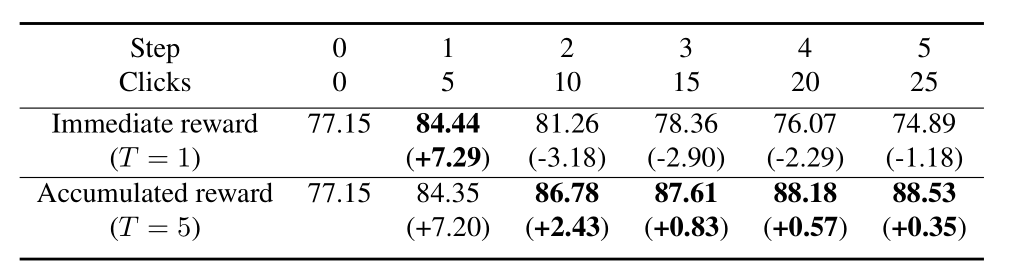表7。累积奖励vs即时奖励
图6显示了预测和提示之间关系的可视化。图6(a)显示了用户交互对预测和提示图的影响。因为数据是3D，我们用click(中间行)和它的两个相邻的切片(两边的行)来显示切片。提示地图上的红色部分是推荐的对象区域。我们发现，所提出的算法可以成功地纠正用户点击点周围的边缘区域(红点)。此外，对相邻片上的相应区域也进行了改进。在图6(b)中，我们在一个包含五个步骤的交互序列中观察到预测和提示图的变化。不会显示用户的单击，因为单击的切片在每个步骤中都是不同的，我们只关注特定切片的更改。利用提示图的提示，IteR-MRL成功地逐步完善了初始预测。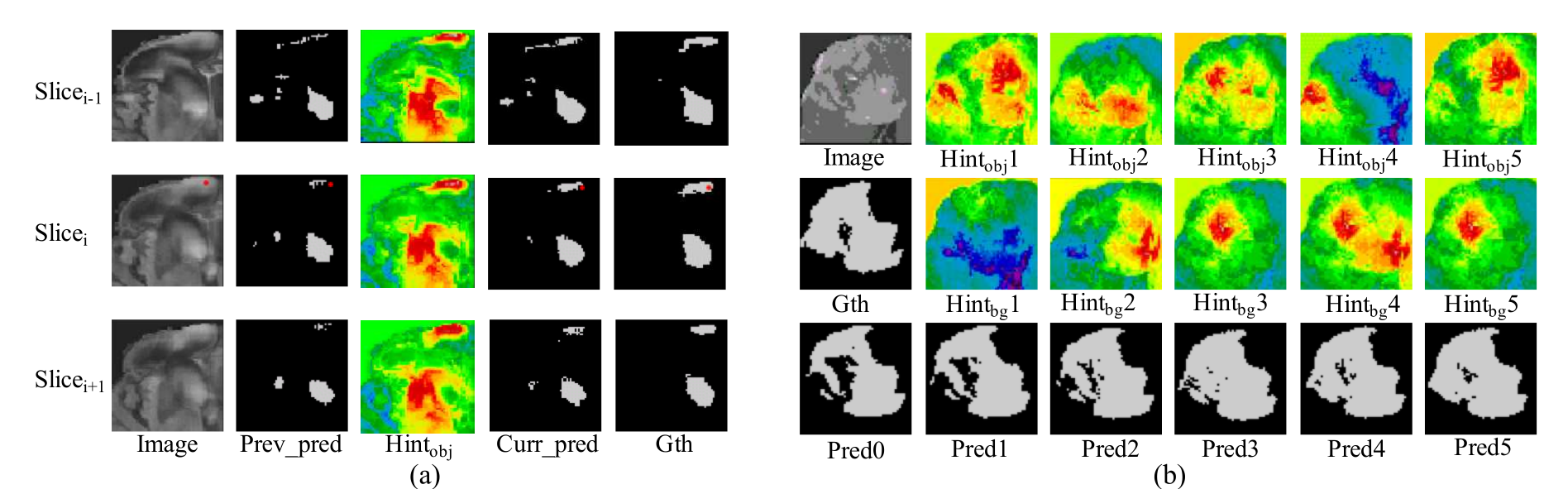图6。预测与提示之间关系的可视化。(a)一次点击的可视化及其对预测和提示地图的影响。此时将显示带有click的切片及其两个相邻的切片。用户单击表示为红点。一排五图构成一组，对应一片切片[图像、先前预测、物体提示图、当前预测、Ground truth]。(b)预测的可视化和每一步的提示图。第一列数字为[图像，地面真实值，初始预测]。然后，每一栏形成一个步骤.
5. Conclusion
本文提出了一种基于多智能体强化学习的三维医学图像迭代精细化交互分割方法。该方法显式地模拟了交互式图像分割任务的动态过程，以便在每次迭代时都能得到快速的分割改进。实验结果表明，该方法比现有方法具有更好的性能，并且对不同的初始分割和数据集具有较强的鲁棒性。
展开全文计算机视觉 强化学习
• 在对医学图像分割三维重建基础理论以及三维重建相关的三维切割技术进行研究和实验的基础上,结合医学图像分割开发工具包ITK(Insight ToolKit)和可视化开发包VTK(Vislual ToolKit),以及ActiveX开发技术,设计了一个...
• 行业分类-物理装置-一种基于深度学习的三维医学图像自动分割方法.zip
• 三维医学图像序列的自动连续分割 非常值得下载文档，值得参考，强烈推荐，下载看看吧，祝你好运，good luck！
• 基于医学图像三维分割技术已经成为现今研究的热点，本文基于ITK,VTK平台下，结合区域生长算法对医学图像进行分割
• 业分类-物理装置-一种三维医学图像中关节软骨组织的自动分割方法.zip
• 摘 要 : 人体组织器官的三维图像分割医学图像分 析和医 疗诊断 的重要 前提, 是医学 图像三 维可视 化的重要 研究 内容。随着 医学成像技术和三维可视化技术的飞速发展, 计算机 辅助诊断成为现实。计算机 技术的...
• 2dmed医学图像分割三维建模软件，适合研究医学图像处理的人们使用
• 针对人体组织器官的三维图像分割医学图像分析和医疗诊断的重要前提，是医学图像三维可视化的重要研究内容。随着医学成像技术和三维可视化技术的飞速发展，计算机辅助诊断成为现实。计算机技术的发展使得医生和研究...
• （最开始接触医学图像分割时写的综述，写的比较幼稚，传上来的时候格式可能有些乱。需要原文的小伙伴可以加我qq...医学图像分割技术的发展不仅影响到医学图像处理中其他相关技术的发展,如可视化、三维重建等，而且在...医学图像处理 卷积神经网络图像分割
• ## 基于深度学习的医学图像分割综述

万次阅读 多人点赞 2018-07-03 10:15:34
医学图像分割技术的发展不仅影响到医学图像处理中其他相关技术的发展，如可视化、三维重建等，而且在生物医学图像的分析中也占有极其重要的地位。近年来,由于深度学习算法在医学图像分割中的应用, 医学图像分割技术...深度学习
• 相当详细的论文资料，有很大的参考价值，是一大牛研究生的毕业论文，概念，代码实现都讲得很清楚了
• 基于GVF主动轮廓模型的医学图像分割及其三维数据场体绘制（硕士论文），对于蛇形算法的研究具有很大的参考价值，仅供大家参考
• 针对进化算法用于图像分割时收敛速度慢、易早熟的...实验结果表明,该算法有效地解决了进化算法收敛速度慢和容易陷入局部极值的问题,而且在分割速度和精度上得到了较大提高,分割效果良好,可以满足医学图像三维重建要求.
• 近年来对图像处理的研究已从二维(2D)向三维(3D)及更高维方向发展。但对3D图像分割的研究目前尚不够深入，仍以基于2D图像的分割方法为主。提出了一种可有效利用空间信息的3D图像自适应分割方法：先进行层间插值、空间...
• 这是专栏《图像分割应用》的第3篇文章，本专栏主要介绍图像分割在各个领域的应用、难点、技术要求等常见问题。肿瘤的分割是医学图像分析领域的一个重要内容，相比较前面提到过的脑区...
• 使用3Dunet进行医学图像分割，讲三维图像进行分割
• 本资源为本人自己学习后整理的一些实用资料，分享给开始入门学习ITK进行医学图像分割的同学，ITK 是一个开放 源码、面向对象的软件系统，提供一个医学图像处理、图像分割与配准的算法平台，对医学图像进行二维、三维...
• 医学图像分割： 根据需要选择一定的特征量或指定特定的测量准则来检测图像中不同区域的一致性，根据检测出来的区域一致性将图像区别成不同区域，从而可以更加方便地进行进一步的分析和理解。 <2>.医学图像分割方法...
• 医学图像分割技术的发展不仅影响到医学图像处理中其他相关技术的发展,如可视化、三维重建等,而且在生物医学图像的分析中也占有极其重要的地位。近年来,由于一些新兴学科在医学图像处理中的应用,医学图像分割技术取得...Snake...